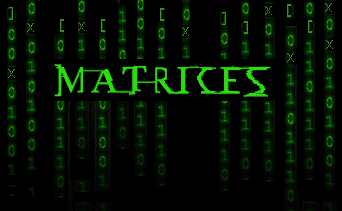Tavish Srivastava — Published On October 29, 2014 and Last Modified On July 26th, 2020I have been using SAS for more than 3 years now. When I started using R, I found a few operations extremely easy. R treats every variable as an object, hence a few operations become very effective on R. For example, multiplication of Vectors and Matrix Calculations. Using R, you can directly reference to any cell in the whole matrix. For instance, you have a matrix with 5 rows and 5 columns. You want to add number 3 to the cell (3,3) . This operation can be done very easily on R, but on SAS, you will have a hard time figuring out the right code. SAS does line by line operation on tables. To reach 3rd row it has to go through 1st and 2nd row. R creates object out of every type of variable and can reach out to every corner of matrix/vector in a single go.

Techniques like Latent Markov Model (LMM), have number of matrix calculations and hence it becomes much simpler to be done on R. But in some cases when you have huge datasets, using SAS becomes imperative. But writing a macro code on SAS for such problems is not only tiring, but also ineffective. SAS Interactive Matrix Language (IML) comes very handy in such matrix calculations. The usage of IML is in no way restricted to coding for LMM. It has a number of uses. Wherever, you see complex matrix calculations, you should always explore IML coding. In this article we will give an introduction to IML coding. But since this topic is huge and I myself am exploring the language, this article will just give you a sweet and simple introduction. We will discuss this subject in more details in coming articles.

### What is Interactive Matrix Language (IML)?

IML is a matrix language similar to MATLAB and Gauss. It enables SAS to do matrix calculation more effectively using many inbuilt matrix functions. It is only capable of handling 2-dimension matrix. There are four main usages of IML codings :

1. It helps to program statistical procedures hard to be coded on SAS. Such procedures are iterative in nature and hence take a lot of time when coded simply using Macros.
2. Perform Matrix operations, for example : calculating inverse, doing matrix multiplications, computing determinant etc.
3. Doing operations on row and column of data sets.
4. Creating cool graphics

In cases where SAS can do the job well, using IML should generally be avoided (as it reserves some extra time creating data structures in the memory).

### Codes to get you started

Let’s start coding on SAS IML with some very basic codes. Here is a code to initialize a simple variable on IML.

The above code creates a table which is shown on the below the code. As you can see a single number is also stored in form of a Matrix. This matrix has only 1 row and 1 column. Let us now create a more comprehensive matrix and learn some simple matrix functions. Following code creates a matrix of 3 rows and 2 columns.

TRANSPOSING A MATRIX :

Following code will enable you to transpose a given matrix :

SIMPLE MATHEMATICAL OPERATOR FOR TWO MATRICES :

Following code will enable you to add up the two matrix you calculated in last section :

OTHER MATRIX OPERATIONS:

We can do a matrix multiplication of a cell-by-cell multiplication using “*” and “#” respectively. Following is an example code for the same:

### End Notes

This article was just an introduction to IML coding. By now you would have realized how easy it becomes to do matrix operations. With such ease doing matrix calculations, IML becomes very handy. IML programming is generally used for any calculations which involves matrices or iterations. In the next article we will see some more advanced matrix functions which can be done using IML.

Did you find the article useful? Have you used IML before?  Do you plan to use IML in any of your business problems? If yes, share with us how IML programming will simplify or enable your analysis.

### If you like what you just read & want to continue your analytics learning, subscribe to our emails, follow us on twitter or like our facebook page.###### Tavish Srivastava

Tavish Srivastava, co-founder and Chief Strategy Officer of Analytics Vidhya, is an IIT Madras graduate and a passionate data-science professional with 8+ years of diverse experience in markets including the US, India and Singapore, domains including Digital Acquisitions, Customer Servicing and Customer Management, and industry including Retail Banking, Credit Cards and Insurance. He is fascinated by the idea of artificial intelligence inspired by human intelligence and enjoys every discussion, theory or even movie related to this idea.